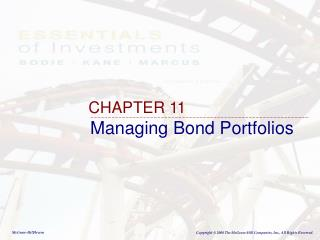# CHAPTER 11 - PowerPoint PPT PresentationDownload PresentationCHAPTER 11

CHAPTER 11Download Presentation## CHAPTER 11

- - - - - - - - - - - - - - - - - - - - - - - - - - - E N D - - - - - - - - - - - - - - - - - - - - - - - - - - -
##### Presentation Transcript

1. CHAPTER 11 Managing Bond Portfolios

2. 11.1 INTEREST RATE RISK

3. Interest Rate Sensitivity • Inverse relationship between price and yield • An increase in a bond’s yield to maturity results in a smaller price decline than the gain associated with a decrease in yield • Long-term bonds tend to be more price sensitive than short-term bonds

4. Interest Rate Sensitivity (cont) • Sensitivity of bond prices to changes in yields increases at a decreasing rate as maturity increases • Interest rate risk is inversely related to bond’s coupon rate • Sensitivity of a bond’s price to a change in its yield is inversely related to the yield to maturity at which the bond currently is selling

5. Figure 11.1 Change in Bond Price as a Function of YTM

6. Duration • A measure of the effective maturity of a bond • The weighted average of the times until each payment is received, with the weights proportional to the present value of the payment • Duration is shorter than maturity for all bonds except zero coupon bonds • Duration is equal to maturity for zero coupon bonds

7. Duration: Calculation t = + Pr ice ( 1 y ) ] w [CF t t T å = ´ D t w t = t 1 = CF Cash Flow for period t t

8. Duration Calculation

9. Figure 11.3 Duration as a Function of Maturity

10. Duration/Price Relationship • Price change is proportional to duration and not to maturity DP/P = -D x [Dy / (1+y)] D* = modified duration D* = D / (1+y) DP/P = - D* x Dy

11. 11.2 PASSIVE BOND MANAGEMENT

12. Immunization • Passive management • Net worth immunization • Target date immunization

13. Figure 11.4 Growth of Invested Funds

14. Figure 11.5 Immunization

15. Cash Flow Matching and Dedication • Automatically immunizes a portfolio from interest rate movements • Cash flow from the bond and the obligation exactly offset each other • Not widely pursued • Sometimes not even possible

16. 11.3 CONVEXITY

17. Limitations of Duration • Duration is only an approximation • Duration asserts that the percentage price change is directly proportional to the change in the bond’s yield • Underestimates the increase in bond prices when yield falls • Overestimates the decline in price when the yield rises

18. Pricing Error from Convexity Price Pricing Error from Convexity Duration Yield

19. Correction for Convexity Modify the pricing equation: D P 1 2 = - ´ D + ´ ´ D D y Convexity ( y ) 2 P Convexity is Equal to: é ù N 1 ( ) CF å + t 2 t ê ú t 2 t ´ + + P (1 y) ( 1 y ) ë û = t 1 Where: CFt is the cash flow (interest and/or principal) at time t.

20. Figure 11.6 Bond Price Convexity

21. Figure 11.7 Convexity of Two Bonds

22. 11.4 ACTIVE BOND MANAGEMENT

23. Swapping Strategies • Substitution swap • Intermarket swap • Rate anticipation swap • Pure yield pickup • Tax swap

24. Horizon Analysis • Analyst selects a particular investment period and predicts bond yields at the end of that period

25. Contingent Immunization • Allow the managers to actively manage until the bond portfolio falls to a threshold level • Once the threshold value is hit the manager must then immunize the portfolio • Active with a floor loss level

26. Figure 11.8 Contingent Immunization